Learn data science and machine learning by building real-world projects on Jovian

## Final project: - Weather Image Classification using CNN in PyTorch.

In :
``````import os
import torch
import torchvision
import tarfile
from torch.utils.data import random_split``````
In :
``project_name='cnn-weather-image-classification'``

### DataSet Used

For this assignmente the folowing dataset was used:

Multi-class Weather Dataset for Image Classification Published: 13-09-2018 | Version 1 | DOI: 10.17632/4drtyfjtfy.1 Contributor: Gbeminiyi Ajayi. Description. Multi-class weather dataset(MWD) for image classification is a valuable dataset used in the research paper entitled “Multi-class weather recognition from still image using heterogeneous ensemble method”. The dataset provides a platform for outdoor weather analysis by extracting various features for recognizing different weather conditions.

https://data.mendeley.com/datasets/4drtyfjtfy/1

The original dataset was modified for having a set of 32x32 images, and the same number of samples for each of four classes considered (cloudy, rain, shune and sunrise). This modified dataset can be downloaded from:

In :
``````from google.colab import drive
drive.mount('/content/gdrive')``````
```Drive already mounted at /content/gdrive; to attempt to forcibly remount, call drive.mount("/content/gdrive", force_remount=True). ```
In :
``! rm -rf ./data``
In :
``````!unrar x -inul "/content/gdrive/MyDrive/data/weather32.rar" "./data/"

``````
In :
``````if (os.path.isdir('./data')):
print("Data OK")``````
```Data OK ```

The dataset is extracted to the directory `data/weather`. It contains 2 folders `train` and `test`, containing the training set ( 800 images) and test set (60 images) respectively. Each of them contains 4 folders, one for each class of images. Let's verify this using `os.listdir`.

In :
``````data_dir = './data/weather'

print(os.listdir(data_dir))
classes = os.listdir(data_dir + "/train")
print(classes)
``````
```['train', 'test'] ['sunrise', 'rain', 'cloudy', 'shine'] ```

Let's look inside a couple of folders, one from the training set and another from the test set. As an exercise, you can verify that that there are an equal number of images for each class, 800 in the training set and 60 in the test set.

In :
``````shine_files = os.listdir(data_dir + "/train/shine")
print('No. of training examples for shine:', len(shine_files))
print(shine_files[:5])``````
```No. of training examples for shine: 200 ['shine59.jpg', 'shine98.jpg', 'shine87.jpg', 'shine125.jpg', 'shine29.jpg'] ```
In :
``````cloudy_files = os.listdir(data_dir + "/test/cloudy")
print('No. of test examples for cloudy:', len(cloudy_files))
print(cloudy_files[:5])``````
```No. of test examples for cloudy: 15 ['cloudy209.jpg', 'cloudy211.jpg', 'cloudy212.jpg', 'cloudy206.jpg', 'cloudy204.jpg'] ```

The above directory structure (one folder per class) is used by many computer vision datasets, and most deep learning libraries provide utilites for working with such datasets. We can use the `ImageFolder` class from `torchvision` to load the data as PyTorch tensors.

In :
``````from torchvision.datasets import ImageFolder
from torchvision.transforms import ToTensor``````
In :
``dataset = ImageFolder(data_dir+'/train', transform=ToTensor())``

Let's look at a sample element from the training dataset. Each element is a tuple, containing a image tensor and a label. Since the data consists of 32x32 px color images with 3 channels (RGB), each image tensor has the shape `(3, 32, 32)`.

In :
``````img, label = dataset
print(img.shape, label)
img
``````
```torch.Size([3, 32, 32]) 0 ```
Out:
``````tensor([[[0.3098, 0.2784, 0.3059,  ..., 0.5216, 0.5020, 0.5882],
[0.4118, 0.4980, 0.5765,  ..., 0.5176, 0.6118, 0.7804],
[0.5216, 0.6549, 0.7412,  ..., 0.5922, 0.7294, 0.9059],
...,
[0.0863, 0.0863, 0.0824,  ..., 0.1882, 0.1882, 0.1882],
[0.0784, 0.0784, 0.0745,  ..., 0.1804, 0.1804, 0.1804],
[0.0706, 0.0706, 0.0706,  ..., 0.1765, 0.1765, 0.1804]],

[[0.3098, 0.2784, 0.3059,  ..., 0.5216, 0.5020, 0.5882],
[0.4118, 0.4980, 0.5765,  ..., 0.5176, 0.6118, 0.7804],
[0.5216, 0.6549, 0.7412,  ..., 0.5922, 0.7294, 0.9059],
...,
[0.0863, 0.0863, 0.0824,  ..., 0.1882, 0.1882, 0.1882],
[0.0784, 0.0784, 0.0745,  ..., 0.1804, 0.1804, 0.1804],
[0.0706, 0.0706, 0.0706,  ..., 0.1765, 0.1765, 0.1804]],

[[0.3098, 0.2784, 0.3059,  ..., 0.5216, 0.5020, 0.5882],
[0.4118, 0.4980, 0.5765,  ..., 0.5176, 0.6118, 0.7804],
[0.5216, 0.6549, 0.7412,  ..., 0.5922, 0.7294, 0.9059],
...,
[0.0863, 0.0863, 0.0824,  ..., 0.1882, 0.1882, 0.1882],
[0.0784, 0.0784, 0.0745,  ..., 0.1804, 0.1804, 0.1804],
[0.0706, 0.0706, 0.0706,  ..., 0.1765, 0.1765, 0.1804]]])``````

The list of classes is stored in the `.classes` property of the dataset. The numeric label for each element corresponds to index of the element's label in the list of classes.

In :
``print(dataset.classes)``
```['cloudy', 'rain', 'shine', 'sunrise'] ```

We can view the image using `matplotlib`, but we need to change the tensor dimensions to `(32,32,3)`. Let's create a helper function to display an image and its label.

In :
``````import matplotlib
import matplotlib.pyplot as plt
%matplotlib inline

matplotlib.rcParams['figure.facecolor'] = '#ffffff'``````
In :
``````
def show_example(img, label):
print('Label: ', dataset.classes[label], "("+str(label)+")")
plt.imshow(img.permute(1, 2, 0))``````

Let's look at a couple of images from the dataset. As you can tell, the 32x32px images are quite difficult to identify, even for the human eye. Try changing the indices below to view different images.

In :
``show_example(*dataset)``
```Label: cloudy (0) ```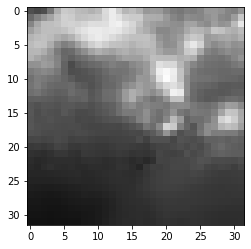In :
``show_example(*dataset)``
```Label: sunrise (3) ```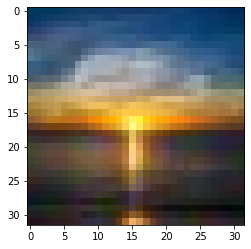In :
``torch.device('cpu')``
Out:
``device(type='cpu')``
In :
``````random_seed = 42
torch.manual_seed(random_seed);
``````
In :
``````val_size = 100
train_size = len(dataset) - val_size

train_ds, val_ds = random_split(dataset, [train_size, val_size])
len(train_ds), len(val_ds)``````
Out:
``(700, 100)``

We can now create data loaders for training and validation, to load the data in batches

In :
``````from torch.utils.data.dataloader import DataLoader

batch_size=35``````
In :
``````train_dl = DataLoader(train_ds, batch_size, shuffle=True, num_workers=4, pin_memory=True)
val_dl = DataLoader(val_ds, batch_size*2, num_workers=4, pin_memory=True)``````

We can look at batches of images from the dataset using the `make_grid` method from `torchvision`. Each time the following code is run, we get a different bach, since the sampler shuffles the indices before creating batches.

In :
``````from torchvision.utils import make_grid

def show_batch(dl):
for images, labels in dl:
fig, ax = plt.subplots(figsize=(12, 6))
ax.set_xticks([]); ax.set_yticks([])
ax.imshow(make_grid(images, nrow=7).permute(1, 2, 0))
break``````
In :
``show_batch(train_dl)``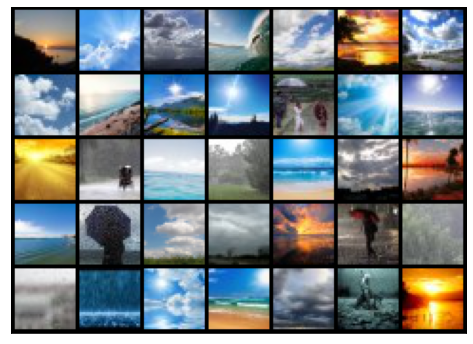### Simple Model of CNN

In :
``````import torch.nn as nn
import torch.nn.functional as F``````
In :
``````simple_model = nn.Sequential(
nn.MaxPool2d(2, 2)
)
``````
In :
``````for images, labels in train_dl:
print('images.shape:', images.shape)
out = simple_model(images)
print('out.shape:', out.shape)
break``````
```images.shape: torch.Size([35, 3, 32, 32]) out.shape: torch.Size([35, 8, 16, 16]) ```

Aplying the simple CNN model to an image it is transformed in a 8 chanel 16x16 pixels image.

## The model

In :
``````class ImageClassificationBase(nn.Module):
def training_step(self, batch):
images, labels = batch
out = self(images)                  # Generate predictions
loss = F.cross_entropy(out, labels) # Calculate loss
return loss

def validation_step(self, batch):
images, labels = batch
out = self(images)                    # Generate predictions
loss = F.cross_entropy(out, labels)   # Calculate loss
acc = accuracy(out, labels)           # Calculate accuracy
return {'val_loss': loss.detach(), 'val_acc': acc}

def validation_epoch_end(self, outputs):
batch_losses = [x['val_loss'] for x in outputs]
epoch_loss = torch.stack(batch_losses).mean()   # Combine losses
batch_accs = [x['val_acc'] for x in outputs]
epoch_acc = torch.stack(batch_accs).mean()      # Combine accuracies
return {'val_loss': epoch_loss.item(), 'val_acc': epoch_acc.item()}

def epoch_end(self, epoch, result):
print("Epoch [{}], train_loss: {:.4f}, val_loss: {:.4f}, val_acc: {:.4f}".format(
epoch, result['train_loss'], result['val_loss'], result['val_acc']))

def accuracy(outputs, labels):
_, preds = torch.max(outputs, dim=1)

We'll use nn.Sequential to chain the layers and activations functions into a single network architecture.

In :
``````class WeatherCnnModel(ImageClassificationBase):
def __init__(self):
super().__init__()
self.network = nn.Sequential(
nn.ReLU(),
nn.ReLU(),
nn.MaxPool2d(2, 2), # output: 64 x 16 x 16

nn.ReLU(),
nn.ReLU(),
nn.MaxPool2d(2, 2), # output: 128 x 8 x 8

nn.ReLU(),
nn.ReLU(),
nn.MaxPool2d(2, 2), # output: 256 x 4 x 4

nn.Flatten(),
nn.Linear(256*4*4, 1024),
nn.ReLU(),
nn.Linear(1024, 512),
nn.ReLU(),
nn.Linear(512, 4))

def forward(self, xb):
return self.network(xb)``````
In :
``````model = WeatherCnnModel()
model
``````
Out:
``````WeatherCnnModel(
(network): Sequential(
(0): Conv2d(3, 32, kernel_size=(3, 3), stride=(1, 1), padding=(1, 1))
(1): ReLU()
(2): Conv2d(32, 64, kernel_size=(3, 3), stride=(1, 1), padding=(1, 1))
(3): ReLU()
(4): MaxPool2d(kernel_size=2, stride=2, padding=0, dilation=1, ceil_mode=False)
(5): Conv2d(64, 128, kernel_size=(3, 3), stride=(1, 1), padding=(1, 1))
(6): ReLU()
(7): Conv2d(128, 128, kernel_size=(3, 3), stride=(1, 1), padding=(1, 1))
(8): ReLU()
(9): MaxPool2d(kernel_size=2, stride=2, padding=0, dilation=1, ceil_mode=False)
(10): Conv2d(128, 256, kernel_size=(3, 3), stride=(1, 1), padding=(1, 1))
(11): ReLU()
(12): Conv2d(256, 256, kernel_size=(3, 3), stride=(1, 1), padding=(1, 1))
(13): ReLU()
(14): MaxPool2d(kernel_size=2, stride=2, padding=0, dilation=1, ceil_mode=False)
(15): Flatten(start_dim=1, end_dim=-1)
(16): Linear(in_features=4096, out_features=1024, bias=True)
(17): ReLU()
(18): Linear(in_features=1024, out_features=512, bias=True)
(19): ReLU()
(20): Linear(in_features=512, out_features=4, bias=True)
)
)``````

Let's verify that the model produces the expected output on a batch of training data. The 4 outputs for each image can be interpreted as probabilities for the 4 target classes (after applying softmax), and the class with the highest probability is chosen as the label predicted by the model for the input image. Check out Part 3 (logistic regression) for a more detailed discussion on interpeting the outputs, applying softmax and identifying the predicted labels.

In :
``````for images, labels in train_dl:
print('images.shape:', images.shape)
out = model(images)
print('out.shape:', out.shape)
print('out:', out)
break``````
```images.shape: torch.Size([35, 3, 32, 32]) out.shape: torch.Size([35, 4]) out: tensor([-0.0157, 0.0333, -0.0447, 0.0146], grad_fn=<SelectBackward>) ```

To seamlessly use a GPU, if one is available, we define a couple of helper functions (get_default_device & to_device) and a helper class DeviceDataLoader to move our model & data to the GPU as required. These are described in more detail in the previous tutorial.

In :
``````def get_default_device():
"""Pick GPU if available, else CPU"""
if torch.cuda.is_available():
else:

def to_device(data, device):
"""Move tensor(s) to chosen device"""
if isinstance(data, (list,tuple)):
return [to_device(x, device) for x in data]
return data.to(device, non_blocking=True)

"""Wrap a dataloader to move data to a device"""
def __init__(self, dl, device):
self.dl = dl
self.device = device

def __iter__(self):
"""Yield a batch of data after moving it to device"""
for b in self.dl:
yield to_device(b, self.device)

def __len__(self):
"""Number of batches"""
return len(self.dl)``````

Based on where you're running this notebook, your default device could be a CPU (torch.device('cpu')) or a GPU (torch.device('cuda'))

In :
``````device = get_default_device()
device
``````
Out:
``device(type='cpu')``

We can now wrap our training and validation data loaders using DeviceDataLoader for automatically transferring batches of data to the GPU (if available), and use to_device to move our model to the GPU (if available).

In :
``````train_dl = DeviceDataLoader(train_dl, device)
to_device(model, device);
``````

### Training the Model

We'll define two functions: `fit` and `evaluate` to train the model using gradient descent and evaluate its performance on the validation set. For a detailed walkthrough of these functions, check out the previous tutorial.

In :
``````@torch.no_grad()
model.eval()
outputs = [model.validation_step(batch) for batch in val_loader]
return model.validation_epoch_end(outputs)

history = []
optimizer = opt_func(model.parameters(), lr)
for epoch in range(epochs):
# Training Phase
model.train()
train_losses = []
loss = model.training_step(batch)
train_losses.append(loss)
loss.backward()
optimizer.step()
# Validation phase
result['train_loss'] = torch.stack(train_losses).mean().item()
model.epoch_end(epoch, result)
history.append(result)
return history``````

Before we begin training, let's instantiate the model once again and see how it performs on the validation set with the initial set of parameters.

In :
``model = to_device(WeatherCnnModel(), device)``
In :
``evaluate(model, val_dl)``
Out:
``{'val_acc': 0.28809523582458496, 'val_loss': 1.384425401687622}``

The initial accuracy is around 28%, which is what one might expect from a randomly intialized model (since it has a 1 in 3 chance of getting a label right by guessing randomly).

We'll use the following hyperparmeters (learning rate, no. of epochs, batch_size etc.) to train our model. As an exercise, you can try changing these to see if you have achieve a higher accuracy in a shorter time.

In :
``````num_epochs = 15
lr = 0.00001``````
In :
``history = fit(num_epochs, lr, model, train_dl, val_dl, opt_func)``
```Epoch , train_loss: 1.3868, val_loss: 1.3844, val_acc: 0.2881 Epoch , train_loss: 1.3862, val_loss: 1.3838, val_acc: 0.2881 Epoch , train_loss: 1.3852, val_loss: 1.3824, val_acc: 0.2881 Epoch , train_loss: 1.3823, val_loss: 1.3777, val_acc: 0.5286 Epoch , train_loss: 1.3742, val_loss: 1.3638, val_acc: 0.5214 Epoch , train_loss: 1.3509, val_loss: 1.3245, val_acc: 0.5905 Epoch , train_loss: 1.2822, val_loss: 1.2080, val_acc: 0.5643 Epoch , train_loss: 1.1169, val_loss: 0.9885, val_acc: 0.6619 Epoch , train_loss: 0.9285, val_loss: 0.8125, val_acc: 0.6476 Epoch , train_loss: 0.8217, val_loss: 0.7514, val_acc: 0.6857 Epoch , train_loss: 0.7777, val_loss: 0.7131, val_acc: 0.7190 Epoch , train_loss: 0.7372, val_loss: 0.6847, val_acc: 0.6810 Epoch , train_loss: 0.7171, val_loss: 0.6606, val_acc: 0.7000 Epoch , train_loss: 0.7007, val_loss: 0.6442, val_acc: 0.7571 Epoch , train_loss: 0.6817, val_loss: 0.6387, val_acc: 0.7333 ```

We can also plot the valdation set accuracies to study how the model improves over time.

In :
``````def plot_accuracies(history):
accuracies = [x['val_acc'] for x in history]
plt.plot(accuracies, '-x')
plt.xlabel('epoch')
plt.ylabel('accuracy')
plt.title('Accuracy vs. No. of epochs');``````
In :
``plot_accuracies(history)``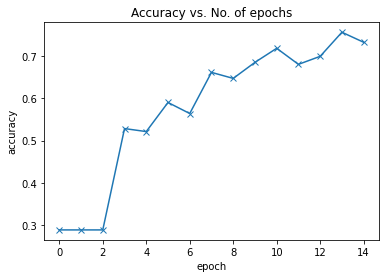Our model reaches an accuracy of around 75%, and by looking at the graph, it seems unlikely that the model will achieve an accuracy higher than 80% even after training for a long time. This suggests that we might need to use a more powerful model to capture the relationship between the images and the labels more accurately. This can be done by adding more convolutional layers to our model, or incrasing the no. of channels in each convolutional layer, or by using regularization techniques.

We can also plot the training and validation losses to study the trend.

In :
``````def plot_losses(history):
train_losses = [x.get('train_loss') for x in history]
val_losses = [x['val_loss'] for x in history]
plt.plot(train_losses, '-bx')
plt.plot(val_losses, '-rx')
plt.xlabel('epoch')
plt.ylabel('loss')
plt.legend(['Training', 'Validation'])
plt.title('Loss vs. No. of epochs');``````
In :
``plot_losses(history)``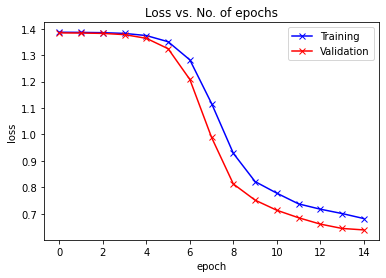### Testing with individual images

While we have been tracking the overall accuracy of a model so far, it's also a good idea to look at model's results on some sample images. Let's test out our model with some images from the predefined test dataset of 10000 images. We begin by creating a test dataset using the `ImageFolder` class.

In :
``test_dataset = ImageFolder(data_dir+'/test', transform=ToTensor())``

Let's define a helper function `predict_image`, which returns the predicted label for a single image tensor.

In :
``````def predict_image(img, model):
# Convert to a batch of 1
xb = to_device(img.unsqueeze(0), device)
# Get predictions from model
yb = model(xb)
# Pick index with highest probability
_, preds  = torch.max(yb, dim=1)
# Retrieve the class label
return dataset.classes[preds.item()]``````
In :
``````img, label = test_dataset
plt.imshow(img.permute(1, 2, 0))
print('Label:', dataset.classes[label], ', Predicted:', predict_image(img, model))``````
```Label: cloudy , Predicted: cloudy ```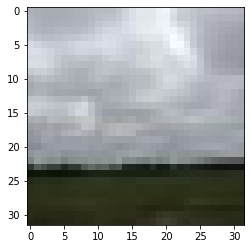In :
``````img, label = test_dataset
plt.imshow(img.permute(1, 2, 0))
print('Label:', dataset.classes[label], ', Predicted:', predict_image(img, model))``````
```Label: shine , Predicted: shine ```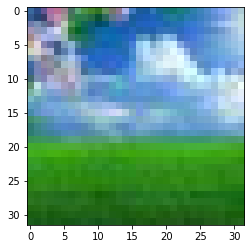In :
``````img, label = test_dataset
plt.imshow(img.permute(1, 2, 0))
print('Label:', dataset.classes[label], ', Predicted:', predict_image(img, model))``````
```Label: sunrise , Predicted: sunrise ```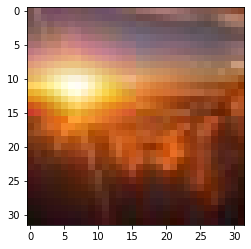Identifying where our model performs poorly can help us improve the model, by collecting more training data, increasing/decreasing the complexity of the model, and changing the hypeparameters.

As a final step, let's also look at the overall loss and accuracy of the model on the test set, and record using `jovian`. We expect these values to be similar to those for the validation set. If not, we might need a better validation set that has similar data and distribution as the test set (which often comes from real world data).

In :
``````test_loader = DeviceDataLoader(DataLoader(test_dataset, batch_size*2), device)
result
``````
Out:
``{'val_acc': 0.8500000238418579, 'val_loss': 0.6343967318534851}``

Since we've trained our model for a long time and achieved a resonable accuracy, it would be a good idea to save the weights of the model to disk, so that we can reuse the model later and avoid retraining from scratch. Here's how you can save the model.

In :
``torch.save(model.state_dict(), 'weather32-cnn.pth')``

The `.state_dict` method returns an `OrderedDict` containing all the weights and bias matrices mapped to the right attributes of the model. To load the model weights, we can redefine the model with the same structure, and use the `.load_state_dict` method.

In :
``model2 = to_device(WeatherCnnModel(), device)``
In :
``model2.load_state_dict(torch.load('weather32-cnn.pth'))``
Out:
``<All keys matched successfully>``

Just as a sanity check, let's verify that this model has the same loss and accuracy on the test set as before.

In :
``evaluate(model2, test_loader)``
Out:
``{'val_acc': 0.8500000238418579, 'val_loss': 0.6343967318534851}``